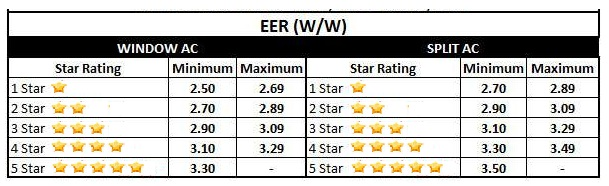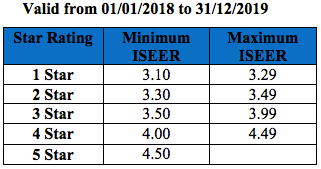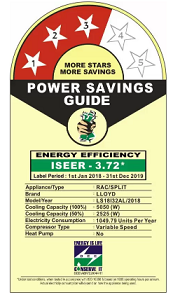# How to Calculate Power Consumption of 1.5 / 1 Ton AC?

It’s chilling summer and most of us might be planning to purchase AC and doing internet research. But the main thing which comes to our mind is the power consumption of AC. As this have a significant effect on our pocket. This post outlines, EER (Energy Efficiency Ratio), ISEER (Indian Seasonal Energy Efficiency Ratio) and the method to calculate the power consumption of 1.5 ton AC in terms of kWh or Unit.

For the sake of calculation of energy / power consumption of AC, we will take 3 STAR, 1.5 ton Split AC as reference. But the method is well applicable for 1 Ton or other tonnage of Window / Split AC. The very first thing is to note down the details provided on the name plate of AC. Following parameters should be noted from the name plate:

• Cooling Capacity
• EER / ISEER
• Inverter AC / Non-Inverter AC

Let’s first discuss each of these parameters in brief.

Cooling Capacity:

Cooling Capacity of AC is defined as the amount of heat energy removed from room. It is given in terms of BTU / hour. BTU stand for British Thermal Unit and it is defined as the amount of heat energy required o raise the temperature of 1 pound of water by 1 degree Fahrenheit. If you calculate this, you will find that

1 BTU = 1055 Joule (Approx)

For 1 Ton AC, the cooling capacity is 12000 BTU/hr. Since 1 BTU = 1055 Joule, therefore , cooling capacity of 1 Ton AC in terms of Watt will be

(12000 x 1055) / 3600 = 3516 Joules / sec.

= 3516 Watt

Similarly, if we consider 1.5 Ton AC, the cooling capacity is 18000 BTU/hr, which means 5275 Watt approx. Thus cooling capacity is fixed by the tonnage of AC.

Energy Efficiency Ratio (EER):

Energy Efficiency Ratio or EER is a parameter which determines the utilization of input power supply to cool the room. Obviously, every consumer should look for better utilization of input power supply as it will help to reduce the electricity consumption and hence bill.

EER of AC is a ratio of Cooling Capacity to the input power supply consumed. Different STAR rating of AC has different value of Energy Efficiency ratio.

EER = Cooling Capacity / Input Power

The unit of EER is thus Watt / Watt.

BEE (Bureau of Energy Efficiency) has set a standard to rate AC on the basis of EER. Refer the table below for relation between STAR rating and EER for Jan 1, 2014 to Dec 31, 2015.From the above table it is clear that if EER lies in between 3.10 to 3.29, the AC will be given 4 STARs. But what does this information mean to a consumer?

The better the EER value, the lower will be the power consumption AC and hence lower will be impact on you pocket. So if you see two 4 STARs ACs, one having EER value of 3.15 and another having 3.25, then you should go for second AC as its EER value is better.

Indian Seasonal Energy Efficiency Ratio (ISEER):

ISEER stands for Indian Seasonal Energy Efficiency Ratio. It is defined as the ratio of total amount of heat energy removed from the indoor air in a year to the total amount of power consumed annually.

ISEER = Cooling Capacity / Annually Power Consumed

The concept of ISEER was launched in India in 24th August 2016. This concept is introduced as the ambient temperature throughout the year varies and hence the cooling capacity of AC also varies to maintain a fixed indoor air temperature. In inverter AC, the tonnage of AC varies with the heat load. This greatly helps in improving the energy efficiency. As the name suggest, it is seasonal energy efficiency ratio.  The better the value of ISEER, the better will be the efficiency of AC.

Based on the concept of ISEER, a new methodology to rate the AC has been adopted. In this method, the ISEER value is calculated for the entire year assuming the temperature profile for the year and 1600 operating hours of AC in a year. Based on the value of ISEER, the STAR rating is given to ACs.### Power Consumption of AC:

If you see the name plate of AC, you will notice that value of ISEER is mentioned. Electricity consumption per year is also mentioned. But it must be noted that, the electricity consumption mentioned on the name plate has been calculated based on certain parameters. The accepted norm of an EER is,  the 27 degree temperature inside the room, the 35 degree temperature outside the room, whereas the humidity is 50%. In the figure, LLOYD Split AC having ISEER Rating of 3.72 has annual power consumption of 1049.79 Units or kWh.The actual power consumption by AC depends on various factors: Room Size, Heat Load in the room, ambient temperature etc. Thus the actual yearly power consumption may vary from what mentioned. But the power consumption mentioned at the name plate may serve as a reference for comparison of various ACs.

### Which AC you should buy?

Obviously, one which consumes less power. As inverter AC is variable tonnage AC. It changes its tonnage as per the ambient and room temperature. Therefore, its energy efficiency is quite good and hence consumes less power. While non-inverter AC always runs at full tonnage and hence consumes more power for the same room cooling.  Therefore, Inverter AC is always recommend. Lets us now decide whether should we buy 3 STAR or 5 STAR AC?

Obviously 5 STAR AC will have more energy efficiency and hence less electricity bill. But the cost of 5 STAR AC is quite higher when compared with 3 STAR AC.

Let us check the impact on our pocket considering fixed and running cost of 5 STAR and 3 STAR AC. Comparison has been made by taking price of Diakin brand 1.5 ton AC. I just found this brand best from performance, durability and maintenance point of view. Above all its cost is quite reasonable.

Rough Cost of 5 Star AC = Rs. 47,000

Rough Cost of 3 Star AC = Rs. 34,000

Saving on Fixed Cost = Rs. 13000 (If you buy 3 STAR Inverter AC)

Annual Power Consumption of 5 STAR Diakin Inverter Split AC (1600 hours in a year)

= 767 Units or kWh

Annual Power Consumption of 3 STAR Diakin Inverter Split AC (1600 hours in a year)

= 1045 Units or kWh

Annual Electricity Bill per Year (assuming Rs. 5 per unit):

Assuming that AC is operated only in summer i.e. 3 months and 8 hours a day = 90×8 = 720 hours.

Power Consumption of 5 STAR Diakin Inverter Split AC for 720 hours

= (767×720) / 1600

= 345 Units or kWh

Power Consuption of 3 STAR Diakin Inverter Split AC for 720 hours

= (1045×720) / 1600

= 470 Units or kWh

Electricity bill for 5 STAR Diakin Inverter Split AC for the entire summer of a year

= Rs. 345×5

= Rs. 1725

Electricity bill for 3 STAR Diakin Inverter Split AC for the entire summer of a year

= Rs. 470×5

= Rs. 2350

Number of years required to save Rs. 13000 electricity bill for 5 STAR AC

= 13000 / 1725

= 7.5 Years

Don’t you think that, in 7.5 years, the AC will also incur maintenance cost? Obviously. So what is the point in purchasing 5 STAR AC for personal household use  if initial cost invested is recovered in 7.5 years. Now, its up to you whether to buy 5 STAR or 3 STAR AC depending on your use. Here is the best ACs.

The calculation shown are indicative. The actual power consumption may vary. The purpose of the article was to convey the information.

### 4 thoughts on “How to Calculate Power Consumption of 1.5 / 1 Ton AC?”

1.Sandeep says:

Very very useful. Thanks

2.Trushen says:

How much watt in 1 ton ac?

3.Sanjay says:

Excellent information. Many thanks

4.pradeep says:

Nicely briefed .. thank you .. Thanks alot sir

This site uses Akismet to reduce spam. Learn how your comment data is processed.